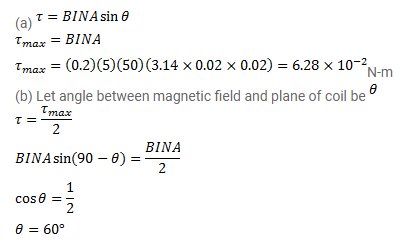# A 50-turn circular coil of radius 2.0cm carrying a current of 5.0A

Question:

A 50 -turn circular coil of radius $2.0 \mathrm{~cm}$ carrying a current of $5.0 \mathrm{~A}$ is rotated in a magnetic field of strength $0.20 \mathrm{~T}$.

(a) What is the maximum torque that acts on the coil?

(b) In a particular position of the coil, the torque acting on it is half of this maximum. What is the angle between the magnetic field and the plane of the coil?

Solution: